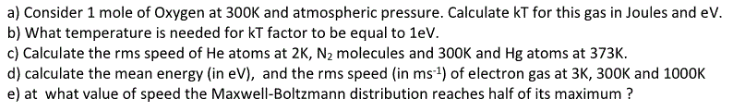Home / Answered Questions / Other / a-consider-1-mole-of-oxygen-at-300k-and-atmospheric-pressure-calculate-kt-for-this-gas-in-joules-and-aw546

# (Solved): A) Consider 1 Mole Of Oxygen At 300K And Atmospheric Pressure. Calculate KT For This Gas In Joules A...a) Consider 1 mole of Oxygen at 300K and atmospheric pressure. Calculate KT for this gas in Joules and eV. b) What temperature is needed for KT factor to be equal to 1eV. c) Calculate the rms speed of He atoms at 2K, N2 molecules and 300K and Hg atoms at 373K d) calculate the mean energy (in eV), and the rms speed (in ms ) of electron gas at 3K, 300K and 1000K e) at what value of speed the Maxwell-Boltzmann distribution reaches half of its maximum ?

We have an Answer from Expert